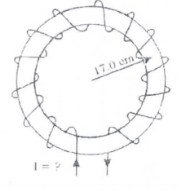# Solution: An ideal toroidal solenoid containing 825 equally spaced coils is shown below. How large must the current I be so that the magnetic field within the coils at a distance of 17 cm from the center is 0.0250 T?

###### Problem

An ideal toroidal solenoid containing 825 equally spaced coils is shown below. How large must the current I be so that the magnetic field within the coils at a distance of 17 cm from the center is 0.0250 T?